Courses
Courses for Kids
Free study material
Free LIVE classes
More

# Carts and Wheels Class 4 Notes CBSE Maths Chapter 8 (Free PDF Download)LIVE
Join Vedantu’s FREE Mastercalss

## Understand Circles with Carts and Wheel Revision Notes and Worksheets

Class 4 Chapter 8 Maths introduces the concept of circles and their various properties in the form of stories and pictorial representations. Students find imbibing these new concepts easier when they have Carts and Wheels revision notes prepared by the expert academicians of Vedantu

They learn what a circle is and how to draw one with a rope. They also learn what a circle is made of. With these revision notes, they also find what the radius, circumference, and diameter of a circle are.

Last updated date: 28th Sep 2023
Total views: 144.9k
Views today: 3.44k

## Access Class - 4 Mathematics Chapter - 8 Carts and Wheels Revision Notes

### Circles

• A circle is a collection of points which are at an equal distance from a certain point in a plane.

• A circle is a round shape figure in a plane.

• Examples - Wheels of a cart, watch, coin, disk, dishes, rings, bracelets, earrings, bangles, buttons etc.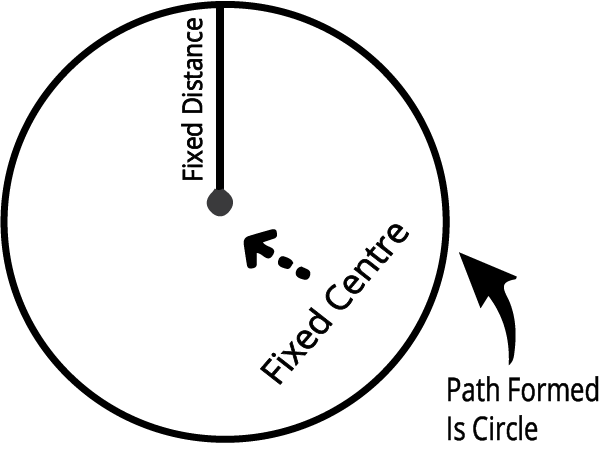Image: Circle

### Parts of a Circle

1. Centre

• A fixed point at an equal distance from every point on the circumference of the circle is known as the centre.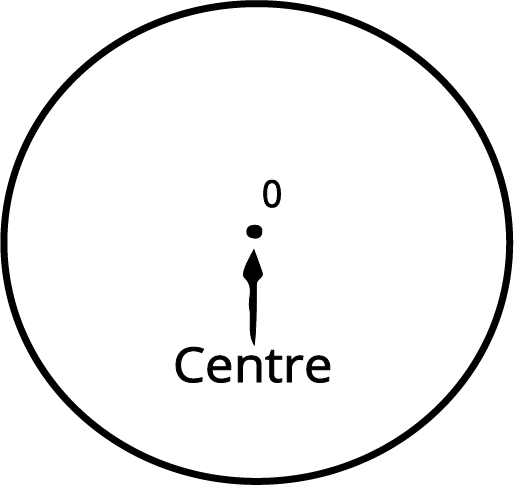Image: Circle with center O

• The line joining the centre and any point on the circumference of the circle is called the radius of the circle.

• The radius is half of the diameter of a circle.

• Radius of the circle =$\frac{\text{diameter of the circle}}{2}$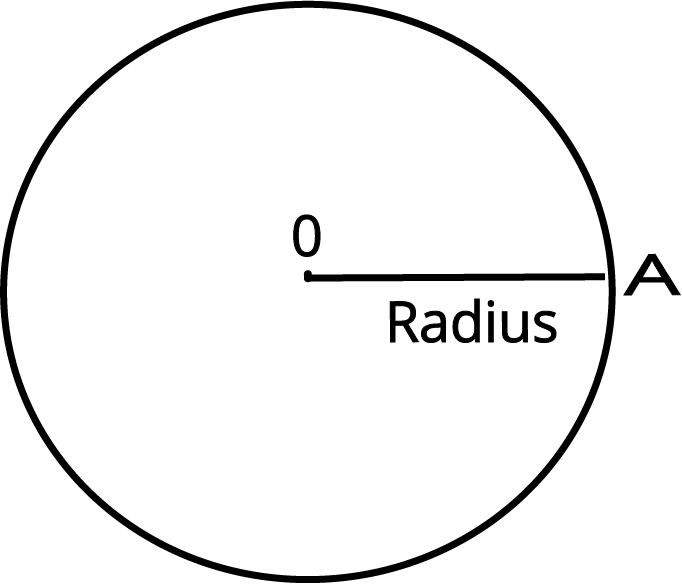### Solved Example

1: Find the radius of a circle if the diameter of the circle is 10cm.

Ans: The radius of a circle is half of the diameter of the circle.

Radius of the circle = $\frac{\text{diameter of the circle}}{2}$

Diameter of the circle = 10 cm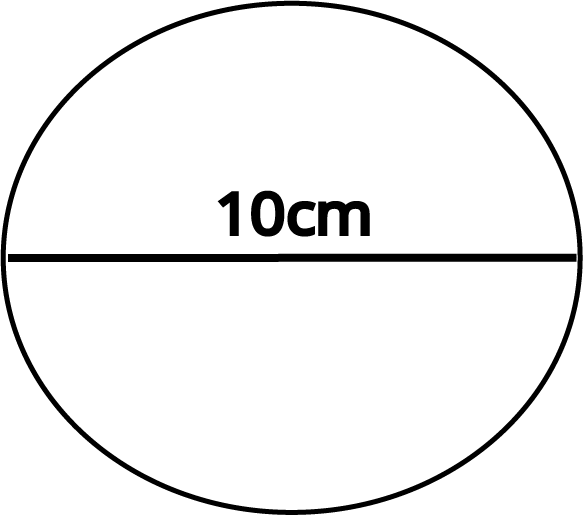Image: Circle with a diameter of 10cm

The radius of a circle = $\frac{\text{10}}{2}$

The radius of a circle = 5 cm

Therefore, the radius of the circle is 5 cm.

2: If the diameter of the circle is 12 cm then find the radius of the circle.

Ans:  The radius of a circle is half of the diameter of the circle.

Radius of the circle = $\frac{\text{diameter of the circle}}{2}$

Diameter of the circle = 12 cm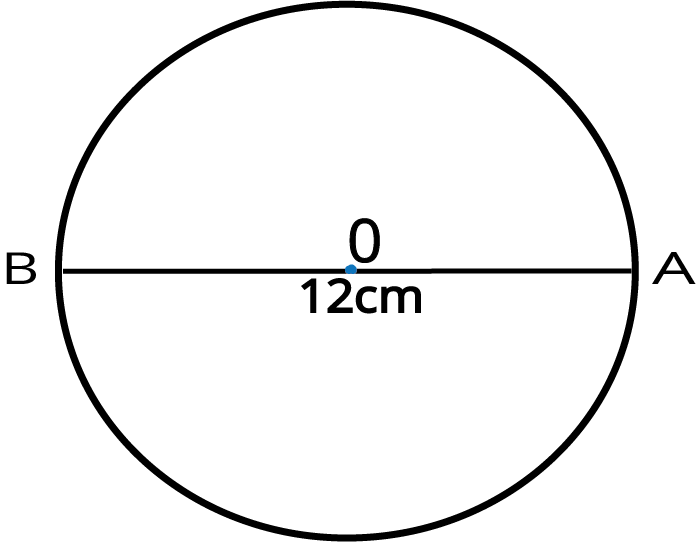Image: Circle with diameter AB = 12cm

The radius of a circle = $\frac{\text{12}}{2}$

The radius of a circle = 6 cm

Therefore, the radius of the circle is 6 cm.

3. Diameter

• The line that joins the two boundary points of a circle and passes through the centre is known as the diameter.

• The diameter is twice the radius of a circle.

Diameter = 2 x Radius of a circle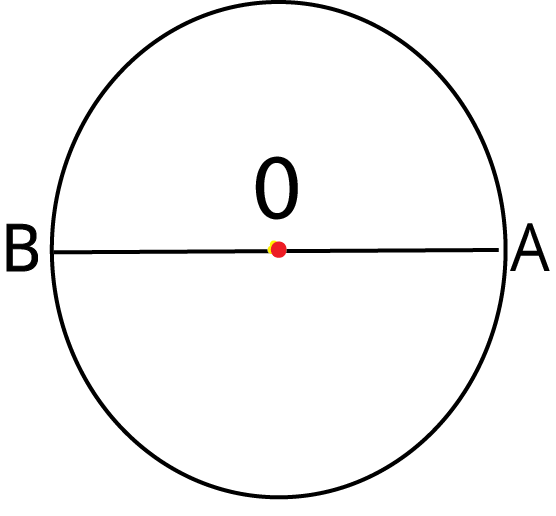Image: Circle with diameter AB

### Solved Example

1: Find the diameter of a circle if the radius of the circle is 10cm?

Ans: The diameter of a circle is twice the radius of a circle.

Diameter =2 x Radius of a circle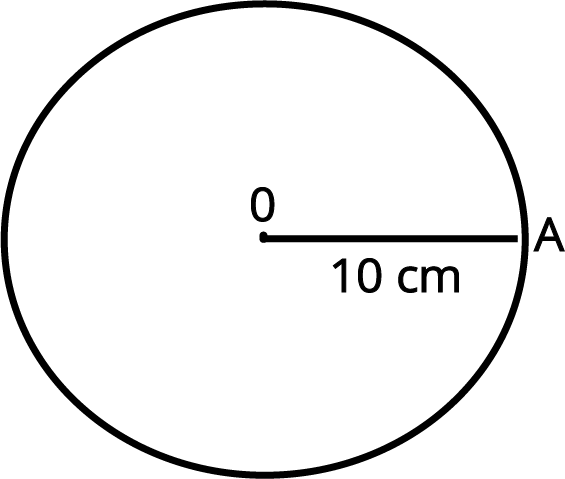Image: Circle with radius OA = 10 cm

Diameter =  2 x 10 cm

Diameter = 20 cm

Therefore, the diameter of a circle is 20 cm.

2: If the radius of a circle is 7 cm then find the diameter of the circle.

Ans: The diameter of a circle is twice the radius of a circle.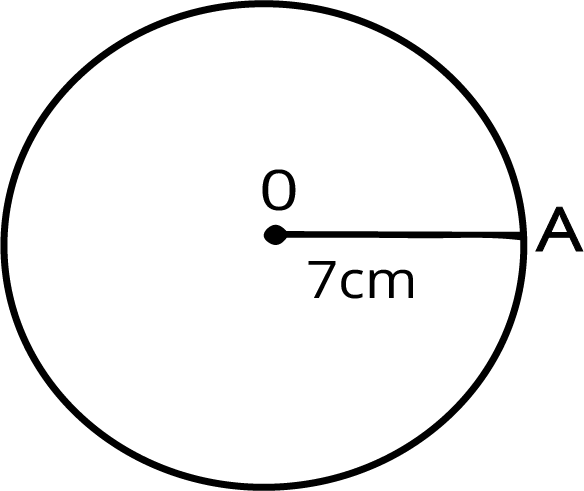Image: Circle with radius OA = 7 cm

Diameter =  2 x Radius of a circle

The diameter of a circle =  2 x7 cm

The diameter of a circle = 14 cm

Therefore, the diameter of a circle is 14 cm.

1. Semicircle

• The diameter of a circle divides the circle into two equal halves and each half is known as a semicircle.

• If two semicircles are combined together then it will be equivalent to a complete circle.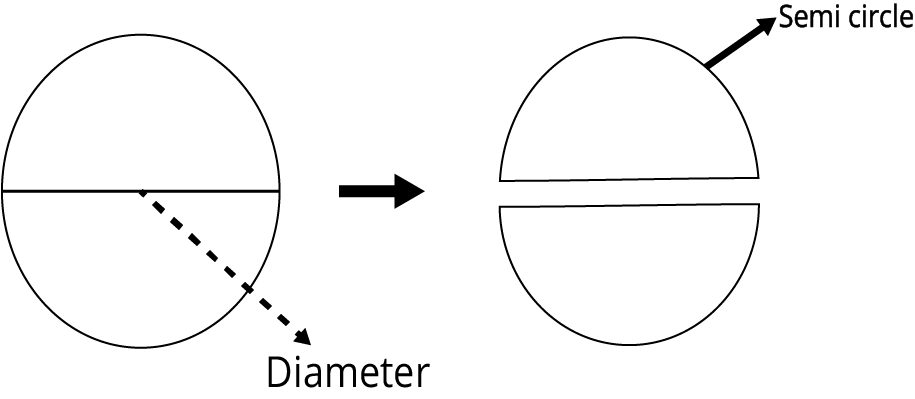Image: Semi circle

1. Circumference

• The circumference is the complete distance of a single round around the circle.

• The circumference is the length of the boundary of a circle.

• Circumference of the circle= 2 x π x Radius of the circle

• Circumference of the circle = π x Diameter of the circle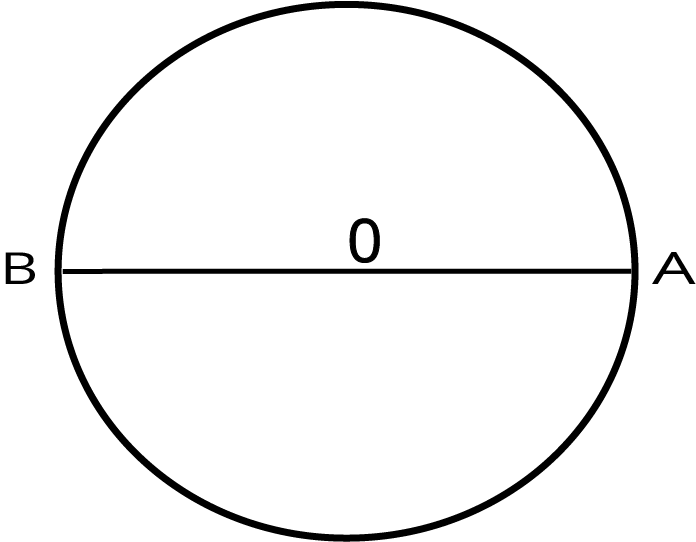Image: Circle with radius OA and diameter AB

### Solved Example

1: If the radius of a circle is 7 cm then find the circumference of the circle.

Ans: The circumference is the length of the boundary of a circle.Image: Circle with radius OA = 7 cm

The circumference of the circle = 2 x π x 7 cm

The circumference of the circle $-2\times \;{\frac{\text{22}}{7}}\;\times 7$ cm

The circumference of the circle= -2 x 22cm

The circumference of the circle = 44 cm

2: Find the circumference of a circle if the diameter of the circle is 10cm?Image: Circle with a diameter of 10cm

Ans: The circumference is the length of the boundary of a circle.

Circumference of a circle =π x Diameter of a circle

Circumference of a circle= ${\frac{\text{22}}{7}}\;\times 10$cm

Circumference of a circle= ${\frac{\text{220}}{7}}$cm

Circumference of a circle = 31.43 cm

### Drawing Circles

To draw a circle of a certain radius we need a compass, a pencil and a scale.

• Step 1: Set the compass needle at 0 on the scale and open the compass equal to the required radius.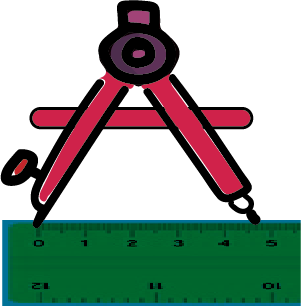• Step 2: Set the compass needle in the centre of a paper sheet.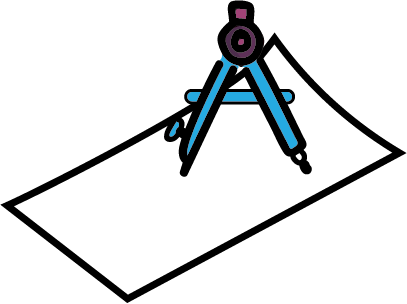Image: Setting the compass needle

• Step 3: Move the pencil around the fixed needle to draw a circle.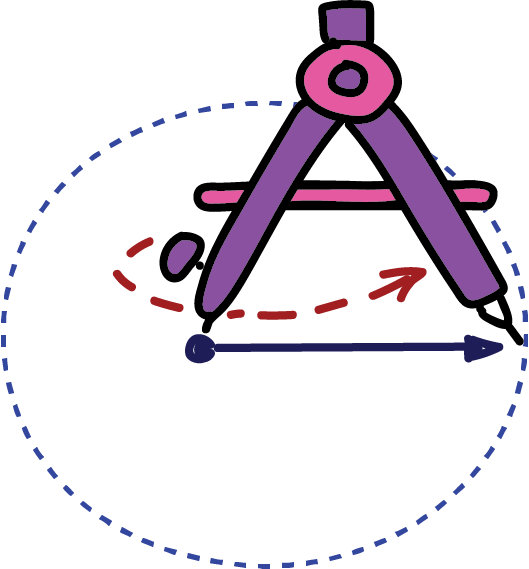Image: Drawing Circle

• Example 1: Draw a circle of 6 cm using a compass and pencil.

Ans: Step 1: Set the compass needle at 0 on the scale and open the compass equal to 6 cm.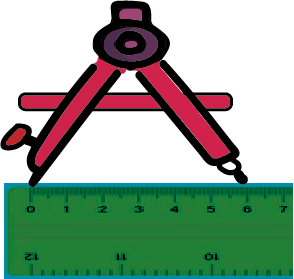Image: Measurement of the radius = 6cm

Step 2: Set the compass needle in the centre of a paper sheet.Image: Setting the compass needle

Step 3: Move the pencil around the fixed needle to draw the required circle.Image: Drawing Circle

### Practice Questions

1. If three friends have different ropes of different lengths. They have made different circles using the ropes which are given below.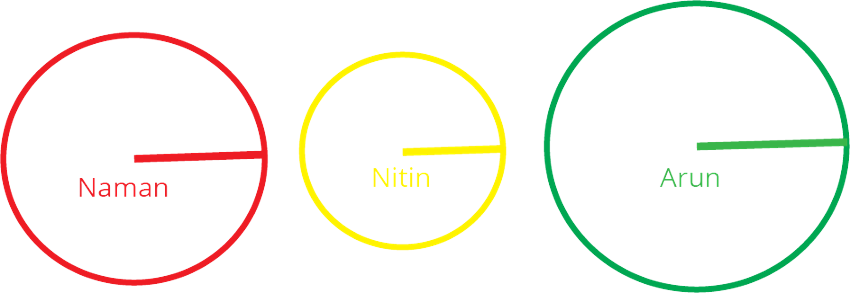(i) which is the smallest circle?

(ii) Which is the largest circle?

Ans:

(i) Nitin’s Circle

(ii) Arun’s Circle

2. If the diameter of a circle is 20 cm then find the radius and circumference of the circle.

Ans: Radius of the circle = 10 cm, Circumference of the circle = 62.86 cm

3. Name the following in the given circle.

(i) Centre

(iii) Diameter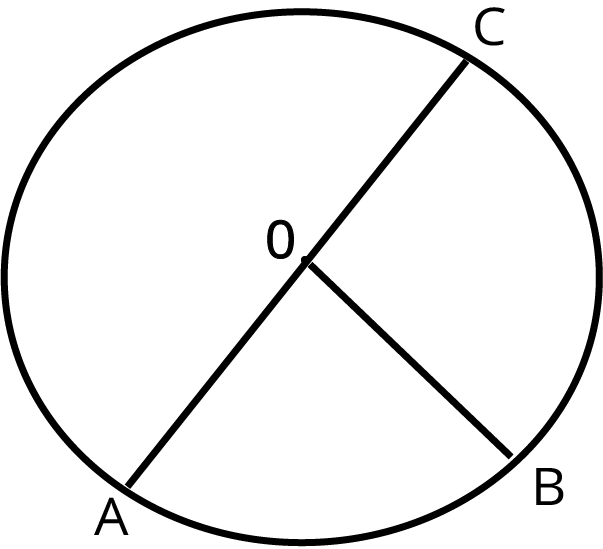Image: Circle with radius OB and diameter AC

Ans :

(i) O

(ii) AO or OB or OC

(iii) AC

## Importance of CBSE Class 4 Maths Chapter 8 Carts and Wheels

The chapter on Carts and Wheels is a unique one in the Class 4 Maths syllabus. This chapter introduces a shape that does not have any straight lines. Previously, students have studied shapes that had sides. Now they will learn what a circle is. Here is why this chapter is very important for children.

• The introduction of curved shapes in this chapter will give new ideas to the students. They will prepare a conceptual base related to circles using daily objects and will become confident to learn advanced geometrical concepts in the future years.

• This chapter explains how a circle can be drawn using just a rope and fixing it on a particular point. It offers a medium of experiential learning for the Class 4 students.

• The Carts and Wheels summary suggests that this chapter teaches how curves can make a symmetrical shape.

• Students will also learn how to draw smaller and bigger circles by simply reducing and increasing a radius.

## Benefits of Vedantu’s Carts and Wheels Class 4 Worksheets and Revision Notes

• Proper explanation of the concepts in simpler language to help you understand faster

• Execution of the exercise problems in a simpler way

• Referring to Carts and Wheels Class 4 worksheets answers will help determine the right ways to solve problems

• Easy and quick preparation before an exam

• Accurate information as per the CBSE Class 4 standards

• Explaining how to make designs with circles using geometric tools

• Learning from the round things around you made easier and more interesting

• Understanding the different parts and features of a circle

## Smooth Studying with Class 4 Maths Chapter 8 Carts and Wheels PDF

The experts have compiled the revision notes and worksheets for this chapter following the NCERT guidelines. You will find the explanation of the circle and its features with definitions easier to comprehend. Preparing Carts and Wheels Class 4 before an exam will become easier.

## FAQs on Carts and Wheels Class 4 Notes CBSE Maths Chapter 8 (Free PDF Download)

1. What is a diameter?

The diameter is the longest chord that fits inside a circle. As per the definition of radius in Class 4th Maths Chapter 8, diameter is equal to two times the radius of a circle.

2. What is the circumference of a circle?

When you perform the activity of making a circle with a rope, you will draw a line on the ground with a stick. The line you draw is the circumference of the circle. It gives the round shape to a circle.

3. Is the ball a circle?

A ball is an object that looks like a circle when you draw it on paper. A circle is a shape. Hence, a ball is called a sphere, a regular round object.